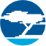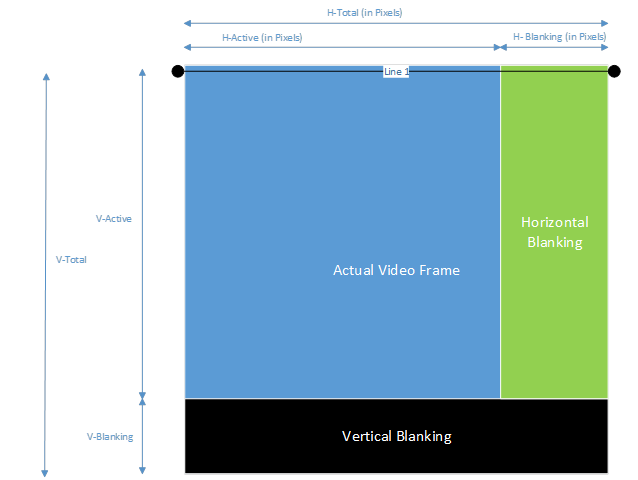cancel
Showing results for
Did you mean:

Analysis of CX3 Video Timing Parameters – KBA226779Employee

Analysis of CX3 Video Timing Parameters – KBA226779

Author:            Version: **

Translation - Japanese: CX3ビデオタイミングパラメーターの分析 - KBA226779 - Community Translated (JA)

Generally, the CX3 MIPI config tool has various video timing parameters which have their own significance. This KBA explains all the video-related parameters of the MIPI block.To understand the timing parameters, you need to introduce a virtual parameter (Pixel clock). Pixel clock is the clock rate at which pixels are transmitting in any video stream. Its value depends upon the video resolution and frames per second (FPS).

Calculate Pixel clock as follows::

If the frames per second is FPS and (H-Active x V-Active) is the video resolution, H-Blank and V-Blank are the blanking time introduced.

Time for one complete frame (i.e., for (H-Total x V-Total) resolution) = 1÷ FPS

Time for one complete horizontal line = (1÷FPS) ÷V-Total (in lines)

Time to send one pixel = (1÷FPS) ÷V-Total (in lines) ÷ H-Total (in pixels)

Then Pixel Clock (in MHz) = (FPS × V-Total × H-Total) ÷ 10^6

Calculate H-Total (in µs): (1÷Pixel Clock)  × H-Total (in Pixels)

To calculate the minimum CSI clock:

The CSI clock is the clock rate at which video data bits enter the MIPI block. Data bits will be transferred at both (rising and falling) edges of the clock as it has DDR support. The number of bits entered at one clock edge is equal to the number of MIPI data lanes selected.

To calculate the CSI spec minimum, you must find the minimum time required by each bit as follows:

Total number of bits in each horizontal line= H-Active × Pixel Depth

Time required by each bit = (H-Active (in Pixels) × Pixel Depth) ÷ H-Total (in µs)

As already mentioned CSI clock is DDR, hence time will become half= (H-Active (in Pixels) × Pixel Depth) ÷  H-Total (in µs) ÷ 2

Bits transferred with each edge of CSI clock= Data Lanes

Therefore, the minimum CSI clock required=  (H-Active (in Pixels) × Pixel Depth) ÷ H-Total (in µs) ÷2 ÷ Data Lanes

To calculate the H-Active time of CSI interface:

The total number of bits in one horizontal line that needs to be transmitted by the MIPI interface= H-Active (in pixels) × Pixel Depth

Bits transferred with each edge of the CSI clock= Data Lanes

The CSI clock has DDR; therefore, the effective clock will be= 2 × CSI_Clock

The time taken to transfer data bits in one horizontal line (H-Active time (in µs)) = [1÷ (2 × CSI_Clock × Data Lanes)] × Pixel Depth × H-Active(in pixels)

To calculate PHY time delay value:

The PHY delay value is the approximate number of ticks taken by CSI RX LP ← → HS clock in “THSPREPARE + (THSZERO /2)” time. This delay value is used to control LP to HS transition. This value is calculated by the tool (see in right bottom corner of the MIPI Receiver Configuration) . It must be manually set using CyU3PMipicsiSetPhyTimeDelay ().

PHY time delay value=   {[THS_Prepare(in ns) + (THS_Zero(in ns)/2)] ÷ [(1÷(CSI RX LP ← → HS Clock(MHz) ×1000)] }-1

Round up this value because the number of ticks cannot be a fraction. 1 is deducted from this value because the range starts from 0.

Version history
Revision #:
3 of 3
Last update:
‎Mar 16, 2021 09:24 PM
Updated by: